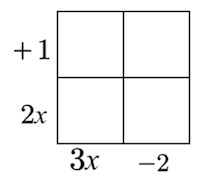Home > CCG > Chapter 5 > Lesson 5.1.2 > Problem5-22

5-22.

Multiply each polynomial. That is, change each product to a sum. Homework Help ✎

1. $(2x+1)(3x-2)$

Draw a generic rectangle. Place the $(2x+1)$ as one side of the rectangle and $(3x−2)$ as another side of the rectangle.Find the area of each part of the rectangle by multiplying $\left(\text{base}\times\text{height}\right)$. Then combine like terms.

$(2x+1)(3x-2)=6x^2-x-2$

1. $(2x+1)(3x^2-2x-5)$

Follow the same steps from part (a), but your generic rectangle will have six boxes instead of four.

1. $(3y-8)(-x+y)$

Follow the same steps from part (a).

1. $(x-3y)(x+3y)$

Follow the same steps from part (a).